## Introduction

A magnetic field fundamentally modifies the transport properties of an electronic conductor by acting on its charge carriers via the Lorenz force. The most prominent magnetotransport effect is the quantum Hall effect in a two-dimensional electron gas. A strong perpendicular magnetic field forces the charge carriers into one-dimensional conduction channels in which they flow along the edges of a sample. At moderate magnetic fields, however, electron trajectories can be understood in a quasiclassical picture where the Lorenz force bends charge carriers into cyclotron orbits. In the bulk this leads to localization, whereas at the boundary charge carriers can propagate via so-called skipping orbits. Magnetic focusing experiments1,2 represent a direct proof of the skipping orbit picture. In such experiments, an increase of conductance is observed if the distance between two contacts is an integer multiple of the diameter of a cyclotron orbit. One condition for the observation of such trajectories is ballistic transport over the relevant device dimensions. This has limited the observation of skipping orbits to the cleanest available semiconductor samples.

Since 2004, graphene as a new two-dimensional conductor has moved into the focus of condensed-matter research and its behaviour in magnetic field has been intensely investigated. The quality of graphene devices has improved over the recent years and ballistic transport over distances of several microns have been demonstrated recently3,4. Since graphene is a gap-less semiconductor, it offers the possibility of creating internal interfaces with opposite charge carrier polarity. These so-called p–n interfaces5,6,7 are formed by local electrostatic gating.

If electrons that propagate via skipping orbits encounter such a p–n interface, they will turn into snake states. These states consist of alternating half circles with opposite chirality and they transport current along the interface. Similar snake states have first been realized in GaAs/AlGaAs two-dimensional electron gases by defining regions of alternating magnetic field direction8. These states share the condition of commensurability (similar to the above-described magnetic focusing experiments) with p–n snake states but they do not propagate along a single and tunable interface. Snake states in graphene p–n junctions were claimed to have been observed in disordered substrate-supported samples9 but the experiment lacked direct evidence for snaking trajectories.

In this article, we investigate ballistic transport across a graphene p–n junction in different magnetic field regimes and identify magneto-conductance oscillations as a direct signature of snake states. These findings are supported by detailed tight-binding simulations that allow us to visualize the alternating cyclotron orbits of the snake states.

## Results

### Evolution of electron trajectories in graphene p–n junction

In Fig. 1a–d, we illustrate schematically how trajectories under increasing perpendicular magnetic field evolve in such a device. Figure 1a describes the low-field situation where transport is still dominated by Fabry–Pérot oscillations with slightly bent trajectories10. As the field is increased, resonant scar states (Fig. 1b) may occur, as observed in semiconductor quantum dots11. At higher fields (Fig. 1c), snake states at the p–n interface govern the electronic properties. Finally (Fig. 1d) the system enters the quantum Hall regime12,13 where transport is dominated by edge states and Landau level mixing at the p–n interface can occur14,15. Even though this article is focusing on snake states, we will discuss the phenomenology of the mentioned magnetic field regimes. By doing so, we present an integral picture of graphene p–n physics in magnetic field.Figure 1: Evolution of electron states in increasing magnetic field and design of a graphene p–n junction.

### Device architecture

Figure 1e shows the design of the measured device and Fig. 1f a scanning electron microscope picture of a similar sample. In a suspended 2 × 2 μm graphene sheet, a p–n junction is formed by applying different voltages on the left (Vleft) and right (Vright) bottom gates, resulting in different charge carrier concentrations nleft and nright. The fabrication follows partly ref. 16, which we combined with a wet transfer process allowing us to align the graphene with the bottom gates. For details, see ref. 17 and Methods.

### Measurements in the Fabry–Pérot and quantum Hall regime

In the following, we characterize the measured device in the zero, low- and high-field regimes. Figure 2a shows a two-dimensional colour map of the electrical conductance G(Vright,Vleft). As soon as a p–n interface is formed, G is lowered drastically. Regular Fabry–Pérot resonances in the left/right cavity are visible as oscillations perpendicular to the zero density line in the left/right cavity (horizontal/vertical white dashed line in Fig. 2a), indicating ballistic transport3,4. The inset in Fig. 2a is a slice along the pp–nn diagonal (blue dashed). We estimate the mobility to beat carrier density n=1.1 × 109 cm−2, which we calculated by a simple parallel plate capacitor model. The mobility is mainly limited by scattering at the contacts3,18.Figure 2: Device characterization in the Fabry–Pérot and in the quantum Hall regime.

In Fig. 2b, we show cuts along the pp–nn diagonal at different magnetic fields B and obtain quantum Hall plateaus at G=G0 × ν, where ν=2,6,10,... is the filling factor12,13 and G0=e2/h is the conductance quantum. Even at fields as low as 60 mT, the ν=2 plateau is visible. The colourscale map in Fig. 2c taken at 200 mT shows that plateaus develop in the unipolar region with conductance values given by the lowest number of edge modes in the left or right cavity, that is, G=G0 × min(νright,νleft)=2, 6, 10 e2/h. This observation compares well to experiments of ref. 14, even though we apply only 0.2 T instead of 4 T. In the bipolar region, the conductance stays well below 2e2/h due to the smoothness of the p–n interface.

In a further step, we study the dispersion of the Fabry–Pérot interference pattern in low magnetic field10. Figure 2d shows the numerical derivative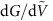as a function of B and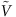, whererepresents the magnitude of gate voltage in the situation of antisymmetric charge density (red dashed line in Fig. 2a, that is, the np–pn diagonal). In this configuration, the device consists of two Fabry–Pérot cavities of equal length L≈0.8 μm. The darkened region where the cyclotron radius Rc<L will be discussed later. In Fig. 2e, a tight-binding transport calculation is shown, which reproduces the measured interference pattern very well (for details see ref. 19 and Methods). We highlight the quality of the measured graphene and the ability of the simulation to capture the complex oscillation pattern of this micron-sized system in the magnetic field. The dispersion of the Fabry–Pérot oscillations can be described by bent electron trajectories such as the one sketched in the inset of Fig. 2e (refs 3, 10). The condition for constructive interference is met if the accumulated phase along such a trajectory is a multiple of 2π (see ‘Methods’). The yellow lines in Fig. 2e are numerical solutions based on such a condition.

### Measurement of snake states

We now discuss the regime where snake states emerge. In Fig. 3a, a snake state at a sharp p–n junction is sketched. Consider a charge carrier trajectory starting at the grey cross with momentum k in −x direction. Due to the magnetic field, the trajectory is bent towards the p–n interface within the cyclotron diameter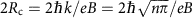. If the trajectory hits the p–n interface, the hole will be transmitted to the n side with high probability due to Klein tunnelling20. At the upper edge of the sample, the snake trajectory scatters at the left side, resulting in a current towards the left contact. At lower n, Rc is reduced. As sketched in Fig. 3b, the snake trajectory scatters to the right at the upper edge, resulting in a net current towards the right contact. With this mechanism, one expects conductance oscillations that depend on B and n, and constant conductance along curves where Rc is constant. In Fig. 3c, we display calculated functions n(B) for constant Rc. Snake states occur once the cyclotron diameter 2Rc is smaller than the sample width W (green dashed curve) and can be described by quasiclassical trajectories as long as Rc is larger than the magnetic length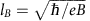(red dashed line). In the regime W>2Rc>lB, additional parabolic lines show the condition for which the number of oscillations in the snake pattern is fixed and commensurate with W, that is, m × 2Rc=W with m=1,2,3,....Figure 3: Parabola-like conductance oscillations as a signature of snake states.

In Fig. 3d, we show the measured conductance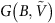(bottom) and its numerical derivative dG/dB (top). The measurement exhibits strong oscillations that follow parabola-like curves. We notice that the oscillations occur on a background of strongly decreasing conductance from G≈6e2/h to G<2e2/h. The steep decrease indicates that the transport becomes dominated by the low-density region close to the p–n interface and this happens when 2Rc<W.

In a real p–n interface, the density does not sharply jump from the p to the n side but evolves smoothly. An electron trajectory in such a smooth p–n interface is sketched in Fig. 3e. Here the density gradient leads to an electric field Ex and its interplay with the perpendicular magnetic field results in E × B drift (here along y), leading to elongated cyclotron orbits. The condition Ry=const. can be studied in the measurement of Fig. 3f, where we show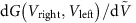at B=120 mT. The measured oscillation pattern follows curves of constant Ex at the p–n interface (obtained from electrostatic simulations) as shown in the inset.

### Tight-binding theory of snake states

So far, we have seen that the oscillation pattern occurs in the regime where snake states are expected (that is, 2Rc<W) and that the oscillations are related to transport along the p–n interface. We now present a quantitative comparison between experiment and theory. Figure 4a shows a quantum transport simulation ofand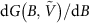on the basis of a scalable tight-binding model19 that fully takes into account the device geometry. The simulation compares very well with the measurement shown in Fig. 3d. The parabola-like patterns are reproduced and a similarly steep decrease of conductance is obtained. In Fig. 4b, a slice following the white dashed line in Fig. 4a is shown. The visibility ΔG/G of the oscillations reaches 30% in theory and experiment and is enabled by the strong Klein collimation at the smooth p–n interface.Figure 4: Tight-binding transport calculation reproducing the experimental results and local current density profiles revealing the snake states.

Next we apply the Keldysh–Green’s function method to extract local current density profiles (see Methods) at high and low conductance along this line. In Fig. 4c, we show the x component of the current density jx, taken at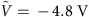(dashed circle in Fig. 4b). The current is injected from the left contact using a small d.c. offset. In the left cavity, a complex resonance pattern appears, given by so-called ‘bubbling’ trajectories21, which are reflected before reaching the p–n interface and do not contribute to current between the contacts. The pattern relevant for transport is located at the p–n interface (dashed line). We observe that jx changes sign along the p–n interface and that the blue and red regions penetrate the dashed line, indicating that transport is dominated by Klein-collimated snake trajectories. As a guide to the eye, we added a curve in Fig. 4d–g that follows the snake state. This is done for differentvalues for which G is maximal/minimal at a fixed magnetic field of 90 mT (coloured circles in Fig. 4b). In Fig. 4d, for example, the current density profile corresponds to a conductance maximum where the current points to the right at the upper edge of the p–n interface. One period is added by changingfrom one maximum to the next. More current density profiles evolving withat fixed B=90 mT are shown in Supplementary Movie 1. By tracing along one of the parabola-like patterns, the current density profile of the snake state stays constant (an example is given in Supplementary Movie 2).

The conductance oscillates as a function of the ratio W/2Ry, the exact snake period corresponding to 4Ry is, however, difficult to determine using quasiclassical trajectories, since current is injected from many points under various angles resulting in a complex cusp structure similar to what was predicted in refs 22, 23, 24, 25. The excellent agreement between measurement and calculated conductance for which we could determine local current density profiles clearly indicates that the oscillations result from snake state trajectories.

There are additional parabola-like structures at lower magnetic field indicated by arrows in Fig. 4a, these structures are, however, less pronounced in the experimental data. Those resonances occur in a regime where scar states (Fig. 1b) would be expected. The parabola-like behaviour indicates that the states are commensurate with a cavity dimension. In the model, the resonances disappear for non-reflective contacts as expected for scar states.

## Discussion

We investigated the magneto-conductance of a ballistic graphene p–n junction in different magnetic field regimes. We have observed resonance patterns occurring in the intermediate quasiclassical regime in experiment and theory, which result from the formation of snake states at the p–n interface. Among many other possibilities, these states can be used to guide electrons on arbitrary paths with a high efficiency even at very low magnetic fields. This could be used to guide electrons away from sample edges to suppress uncontrolled momentum or spin scattering. The directional scattering at the sample boundaries could be used to implement multi-terminal switches24,26. Furthermore, the similarity between Andreev reflection and Klein tunnelling is stressed in theory27 leading to a correspondence of snake states and Andreev edge states which are of theoretical28 and experimental29 interest. Our work points out that snake states are highly tunable and occur at low fields and that ballistic graphene p–n junctions in a magnetic field reveal novel and intriguing phenomena.

## Methods

### Experimental methods

High-quality graphene is obtained by in situ current annealing30. All the measurements were performed in a variable temperature Helium cryostat with a base temperature of 1.5 K. We measured differential conductance G=dI/dV by standard lock-in technique applying an a.c. voltage of 0.1 mV at 77 Hz.

For the quantum Hall data of Fig. 2b,c, we subtracted a contact resistance of 1.2 kΩ.

We extracted the cavity length L used in Fig. 2d,e from the spacing Δn between resonant Fabry–Pérot peaks in the bipolar situation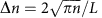and obtained L≈0.8 μm.

### Simulation methods

Real-space Green’s function method in the tight-binding framework using a scaled graphene Hamiltonian19 is applied to simulate ballistic quantum transport in the present device, taking into account the realistic on-site energy profile obtained by three-dimensional electrostatic simulation for the self-partial capacitances of the bottom gates. All the presented conductance simulations are obtained by calculating the transmission function at zero temperature, combined with the contact resistance 1.2 kΩ. Local current densities are imaged by applying the Keldysh–Green’s function method in the linear response regime31 on the basis of the same model Hamiltonian used for conductance simulation. At each lattice site n, the bond charge current density Jn=∑menmJnm› is computed, where the summation runs over all the sites m nearest to n, enm is the unit vector pointing from n to m, and ‹Jnm› is the quantum statistical average of the bond charge current operator Jnm (ref. 32). After computing for each site, the position-dependent current density profile J(x,y)=[jx(x,y),jy(x,y)] is imaged. In Fig. 4c–g, the x component jx(x,y) is shown.

The low-field Fabry–Pérot interference contours sketched in Fig. 2e are numerically obtained from solving the resonance condition ΔΦ=2, arising from the path difference between the directly transmitted and twice reflected trajectories within the p cavity as sketched in the inset of Fig. 2e, which is found to be the major interference contribution. For such a simplified model, the phase difference is given by ΔΦ=ΦWKB+ΦAB+ΦBerry+Φ0, where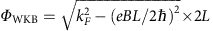is the kinetic WKB-phase, ΦAB=δA × eB/ is the Aharanov–Bohm phase due to the flux enclosed by the bent orbit segments,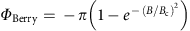is the Berry phase, and Φ0=π is a constant phase due to reflections off the two p–n junction interfaces of the p cavity (smooth at the middle and sharper at the contact side). Here kF is the numerical average of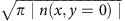within the p cavity. The cavity size L is numerically determined and is about half of the flake length L/2=840 nm. The loop area is given by δA=Rc2(φ−sin φ) with φ=2 arcsin (L/2Rc) and Rc= kF/eB. The form of the Berry phase follows from the consideration of ref. 33 with the critical field estimated by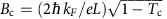, where the critical transmission value Tc is a parameter close to one and does not significantly influence the shape of the contours; Tc=0.95 is chosen. The contours sketched in Fig. 2e correspond to j=1,2,,8.